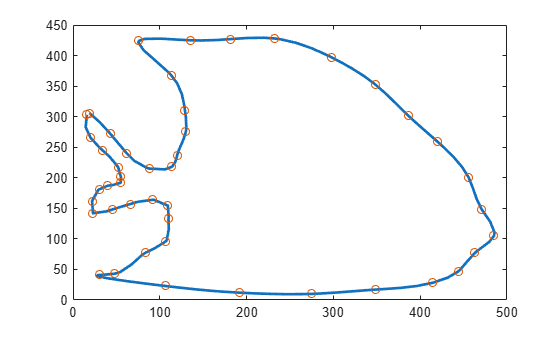# Vector-Valued Functions

Curve Fitting Toolbox™ supports vector-valued splines. For example, if you want a spline curve through given planar points $\left(x\left(i\right),y\left(i\right)\right),i=1,...,n$, then the following code defines some data and then creates and plots such a spline curve, using chord-length parameterization and cubic spline interpolation with the not-a-knot end condition.

```x = [19 43 62 88 114 120 130 129 113 76 135 182 232 298 ... 348 386 420 456 471 485 463 444 414 348 275 192 106 ... 30 48 83 107 110 109 92 66 45 23 22 30 40 55 55 52 34 20 16]; y = [306 272 240 215 218 237 275 310 368 424 425 427 428 ... 397 353 302 259 200 148 105 77 47 28 17 10 12 23 41 43 ... 77 96 133 155 164 157 148 142 162 181 187 192 202 217 245 266 303]; xy = [x;y]; df = diff(xy,1,2); t = cumsum([0,sqrt([1 1]*(df.*df))]); cv = csapi(t,xy); fnplt(cv) hold on plot(x,y,"o") hold off```If you then wanted to know the area enclosed by this curve, you would want to evaluate the integral $\int y\left(t\right)dx\left(t\right)=\int y\left(t\right)Dx\left(t\right)dt$, with $\left(x\left(t\right),y\left(t\right)\right)$ the point on the curve corresponding to the parameter value t. For the spline curve in `cv` just constructed, this can be done exactly in one (somewhat complicated) command:

```area = diff(fnval(fnint( ... fncmb(fncmb(cv,[0 1]),"*",fnder(fncmb(cv,[1 0])))), ... fnbrk(cv,"interval")));```

To explain, `y = fncmb(cv,[0 1])` picks out the second component of the curve in `cv`, `Dx = fnder(fncmb(cv,[1 0]))` provides the derivative of the first component, and `yDx = fncmb(y,"*",Dx)` constructs their pointwise product. Then `IyDx = fnint(yDx)` constructs the indefinite integral of `yDx` and, finally, `diff(fnval(IyDx,fnbrk(cv,"interval")))` evaluates that indefinite integral at the endpoints of the basic interval and then takes the difference of the second from the first value, thus getting the definite integral of `yDx` over its basic interval. Depending on whether the enclosed area is to the right or to the left as the curve point travels with increasing parameter, the resulting number is either positive or negative.

Further, all the values `Y` (if any) for which the point `(X,Y)` lies on the spline curve in `cv` just constructed can be obtained by the following (somewhat complicated) command:

```X = 250; % Define a value of X Y = fnval(fncmb(cv,[0 1]), ... mean(fnzeros(fncmb(fncmb(cv,[1 0]),"-",X))))```
```Y = 1×2 423.4902 9.4812 ```

To explain: `x = fncmb(cv,[1 0])` picks out the first component of the curve in `cv`; `xmX = fncmb(x,"-",X)` translates that component by `X`; `t = mean(fnzeros(xmX))` provides all the parameter values for which `xmX` is zero, that is, for which the first component of the curve equals `X`; `y = fncmb(cv,[0,1])` picks out the second component of the curve in `cv`; and, finally, `Y = fnval(y,t)` evaluates that second component at those parameter sites at which the first component of the curve in `cv` equals `X`.

As another example of the use of vector-valued functions, suppose that you have solved the equations of motion of a particle in some specified force field in the plane, obtaining, at discrete times ${t}_{j}=t\left(j\right),j=1:n$, the position $\left(x\left({t}_{j}\right),y\left({t}_{j}\right)\right)$ as well as the velocity $\left(\underset{}{\overset{˙}{x}}\left({t}_{j}\right),\underset{}{\overset{˙}{y}}\left({t}_{j}\right)\right)$ stored in the 4-vector $z\left(:,j\right)$, as you would if, in the standard way, you had solved the equivalent first-order system numerically. Then the following statement, which uses cubic Hermite interpolation, will produce a plot of the particle path: `fnplt(spapi(augknt(t,4,2),t,reshape(z,2,2*n)))`.